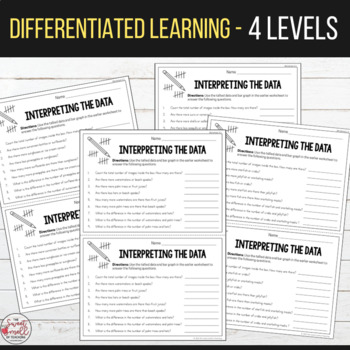# Summer Graphing and Interpreting Data - Differentiated WorksheetsSubject
Resource Type
File Type

PDF

(22 MB|20 pages)
Product Rating
Standards
• Product Description
• StandardsNEW

Summer Graphing & Interpreting Data - Differentiated Worksheets

Students will use their prior knowledge of addition, subtraction and graphing to answer the tally/graph and interpreting data worksheets.

The goal of the activity will be to count and color the images in the main box. They will then tally the total number for each different image. Finally, they will use this information to complete a bar graph and answer questions on a worksheet slip.

This product includes:

- Resource instructions

- 4 tally/graph worksheets

- 4 interpreting data worksheet slips

Level 1

Worksheet 1.1: Count, tally and graph (total number of images within 40).

Worksheet 1.2: Interpreting data from worksheet 1.1.

Level 2

Worksheet 2.1: Count, tally and graph (total number of images within 40).

Worksheet 2.2: Interpreting data from worksheet 2.1.

Level 3

Worksheet 3.1: Count, tally and graph total number of images within 45).

Worksheet 3.2: Interpreting data from worksheet 3.1

Level 4

Worksheet 4.1: Count, tally and graph (total number of images within 50).

Worksheet 4.2: Interpreting data from worksheet 4.1.

Looking for resources involving place value? You may also be interested in:

If you like this product, you may also be interested in these resources:

Operations & Algebraic Thinking

Number & Operations - Fractions

Measurement & Data

Attend to precision. Mathematically proficient students try to communicate precisely to others. They try to use clear definitions in discussion with others and in their own reasoning. They state the meaning of the symbols they choose, including using the equal sign consistently and appropriately. They are careful about specifying units of measure, and labeling axes to clarify the correspondence with quantities in a problem. They calculate accurately and efficiently, express numerical answers with a degree of precision appropriate for the problem context. In the elementary grades, students give carefully formulated explanations to each other. By the time they reach high school they have learned to examine claims and make explicit use of definitions.
Use appropriate tools strategically. Mathematically proficient students consider the available tools when solving a mathematical problem. These tools might include pencil and paper, concrete models, a ruler, a protractor, a calculator, a spreadsheet, a computer algebra system, a statistical package, or dynamic geometry software. Proficient students are sufficiently familiar with tools appropriate for their grade or course to make sound decisions about when each of these tools might be helpful, recognizing both the insight to be gained and their limitations. For example, mathematically proficient high school students analyze graphs of functions and solutions generated using a graphing calculator. They detect possible errors by strategically using estimation and other mathematical knowledge. When making mathematical models, they know that technology can enable them to visualize the results of varying assumptions, explore consequences, and compare predictions with data. Mathematically proficient students at various grade levels are able to identify relevant external mathematical resources, such as digital content located on a website, and use them to pose or solve problems. They are able to use technological tools to explore and deepen their understanding of concepts.
Reason abstractly and quantitatively. Mathematically proficient students make sense of quantities and their relationships in problem situations. They bring two complementary abilities to bear on problems involving quantitative relationships: the ability to decontextualize-to abstract a given situation and represent it symbolically and manipulate the representing symbols as if they have a life of their own, without necessarily attending to their referents-and the ability to contextualize, to pause as needed during the manipulation process in order to probe into the referents for the symbols involved. Quantitative reasoning entails habits of creating a coherent representation of the problem at hand; considering the units involved; attending to the meaning of quantities, not just how to compute them; and knowing and flexibly using different properties of operations and objects.
Make sense of problems and persevere in solving them. Mathematically proficient students start by explaining to themselves the meaning of a problem and looking for entry points to its solution. They analyze givens, constraints, relationships, and goals. They make conjectures about the form and meaning of the solution and plan a solution pathway rather than simply jumping into a solution attempt. They consider analogous problems, and try special cases and simpler forms of the original problem in order to gain insight into its solution. They monitor and evaluate their progress and change course if necessary. Older students might, depending on the context of the problem, transform algebraic expressions or change the viewing window on their graphing calculator to get the information they need. Mathematically proficient students can explain correspondences between equations, verbal descriptions, tables, and graphs or draw diagrams of important features and relationships, graph data, and search for regularity or trends. Younger students might rely on using concrete objects or pictures to help conceptualize and solve a problem. Mathematically proficient students check their answers to problems using a different method, and they continually ask themselves, "Does this make sense?" They can understand the approaches of others to solving complex problems and identify correspondences between different approaches.
Fluently add and subtract within 20 using mental strategies. By end of Grade 2, know from memory all sums of two one-digit numbers.
Total Pages
20 pages
Included
Teaching Duration
1 Week
Report this Resource to TpT
Reported resources will be reviewed by our team. Report this resource to let us know if this resource violates TpT’s content guidelines.